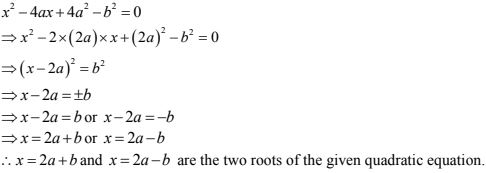# RD Sharma Solutions Chapter 8 Quadratic Equations Exercise 8.4 Class 10 MathsChapter Name RD Sharma Chapter 8 Quadratic Equations Book Name RD Sharma Mathematics for Class 10 Other Exercises Exercise 8.1Exercise 8.2Exercise 8.3Exercise 8.5Exercise 8.6 Related Study NCERT Solutions for Class 10 Maths

### Exercise 8.4 Solutions

Find the roots of the following quadratic equations (if they exist) by the method of completing the square.

1. x2 + 4√2 x + 6 = 0

Solution

We have,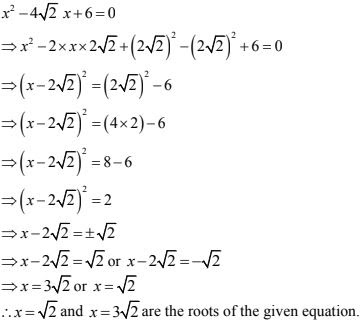2. 2x2 - 7x + 3 = 0

Solution

We have,
2x2 - 7x + 3 = 0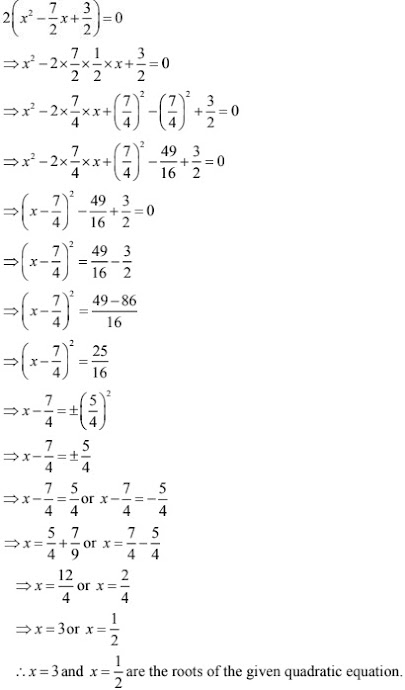3. 3x2 + 11x + 10 = 0

Solution

We have,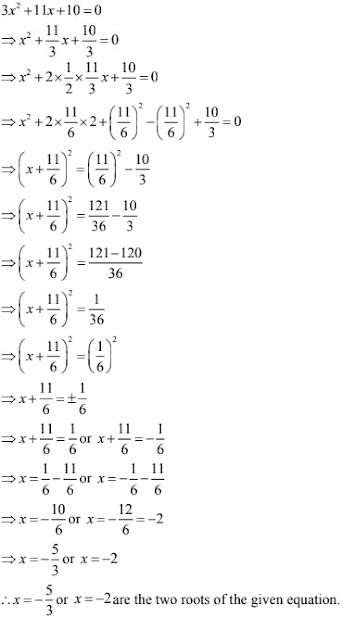4. 2x2 + x - 4 = 0
Solution
2x2 + x - 4 = 0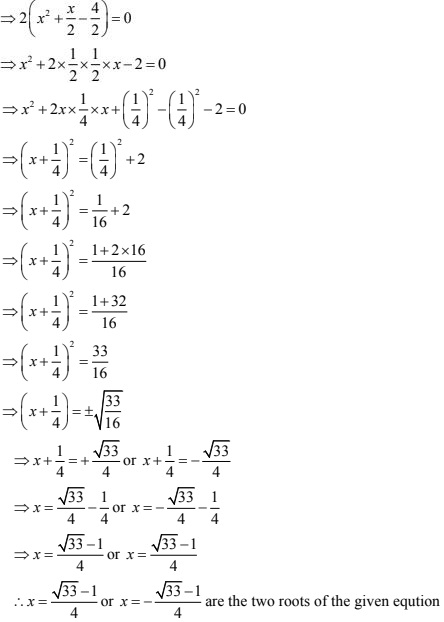5. 2x2 + x + 4 = 0
Solution
We have,
2x2 + x + 4 = 0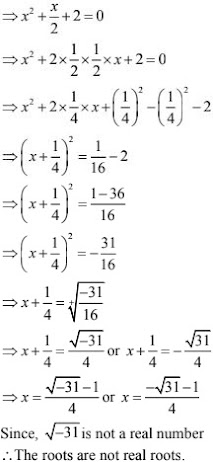6. 4x2 + 4√3 x + 3 = 0
Solution
We have,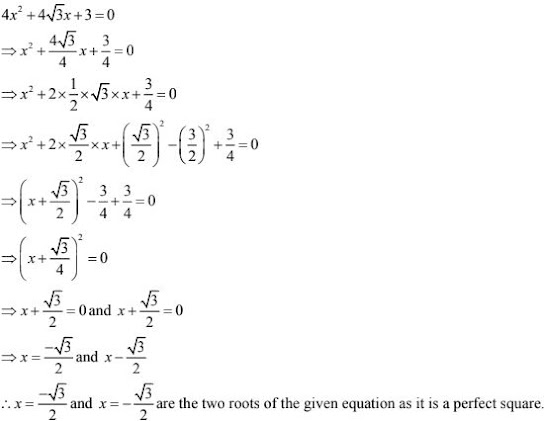7. √2x2 - 3x - 2√2 = 0
Solution
√2x2 - 3x - 2√2 = 0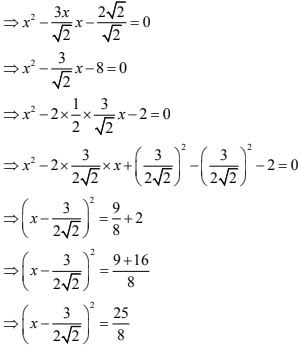8. √3x2 + 10x + 7√3 = 0
Solution
We have
√3x2 + 10x + 7√3 = 0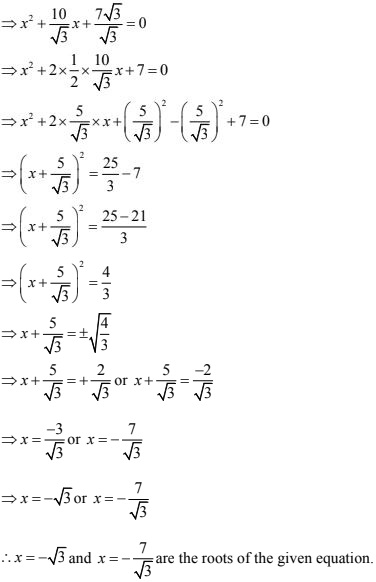9. x2 - (√2 + 1)x + √2 = 0
Solution
We have,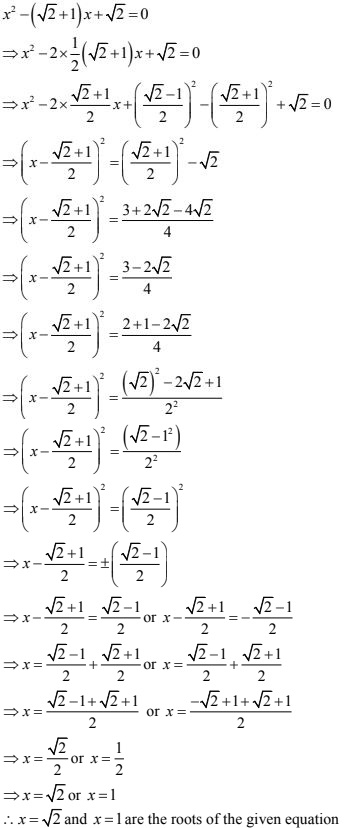10. x2 - 4ax + 4a2 - b2 = 0
Solution
We have,